# RD Sharma Solutions Class 12 Algebra Of Vectors Exercise 23.5

RD Sharma Solutions for Class 12 Maths Chapter 23 Algebra of Vectors Exercise 23.5 are enclosed below to help students outshine in their board exams. The solutions are curated in a manner that every student can understand and attain a firm grip over the concepts. The PDF of RD Sharma Solutions for Class 12 Maths for this exercise can be effortlessly downloaded from the link given below.

## Download PDF of Rd Sharma Solution for Class 12 Maths Chapter 23 Exercise 5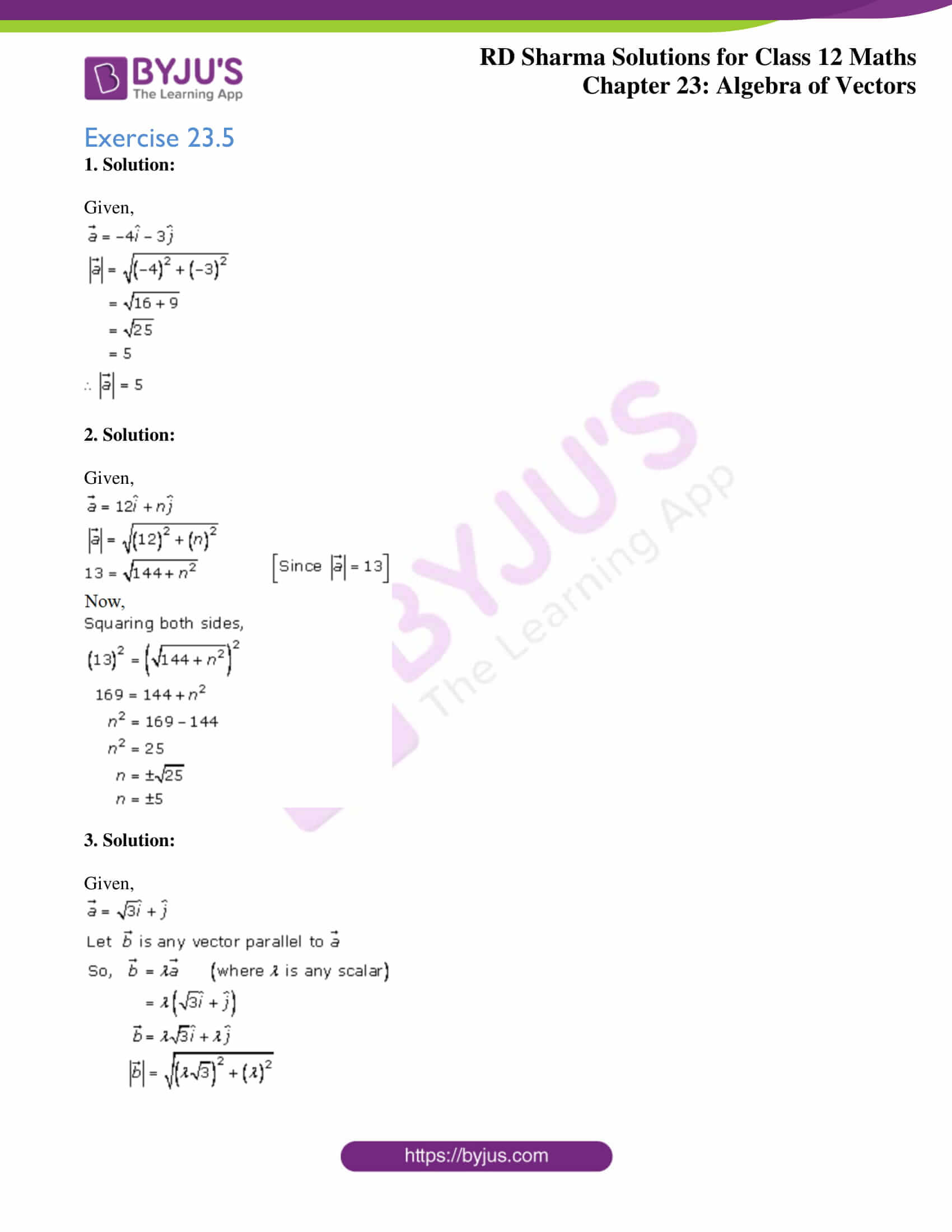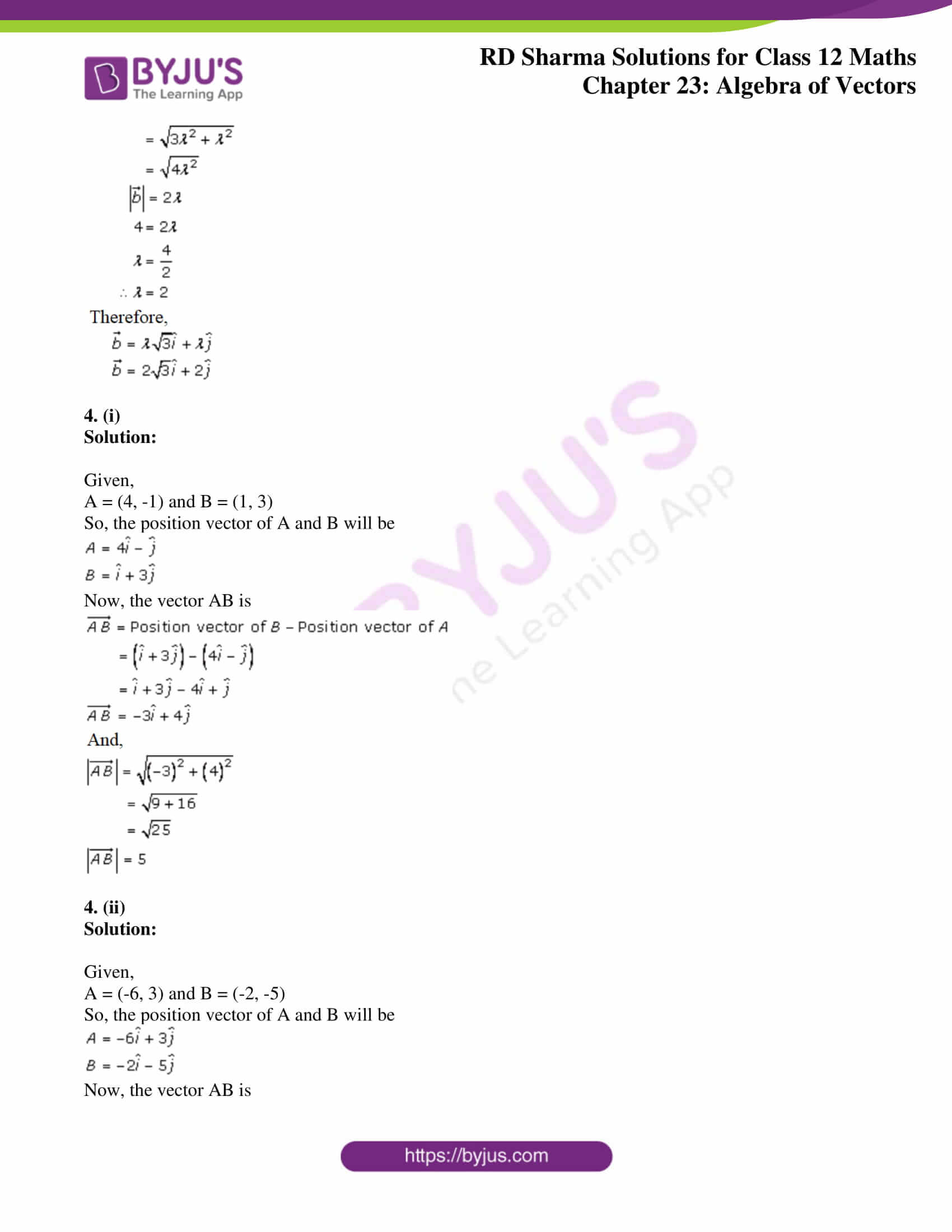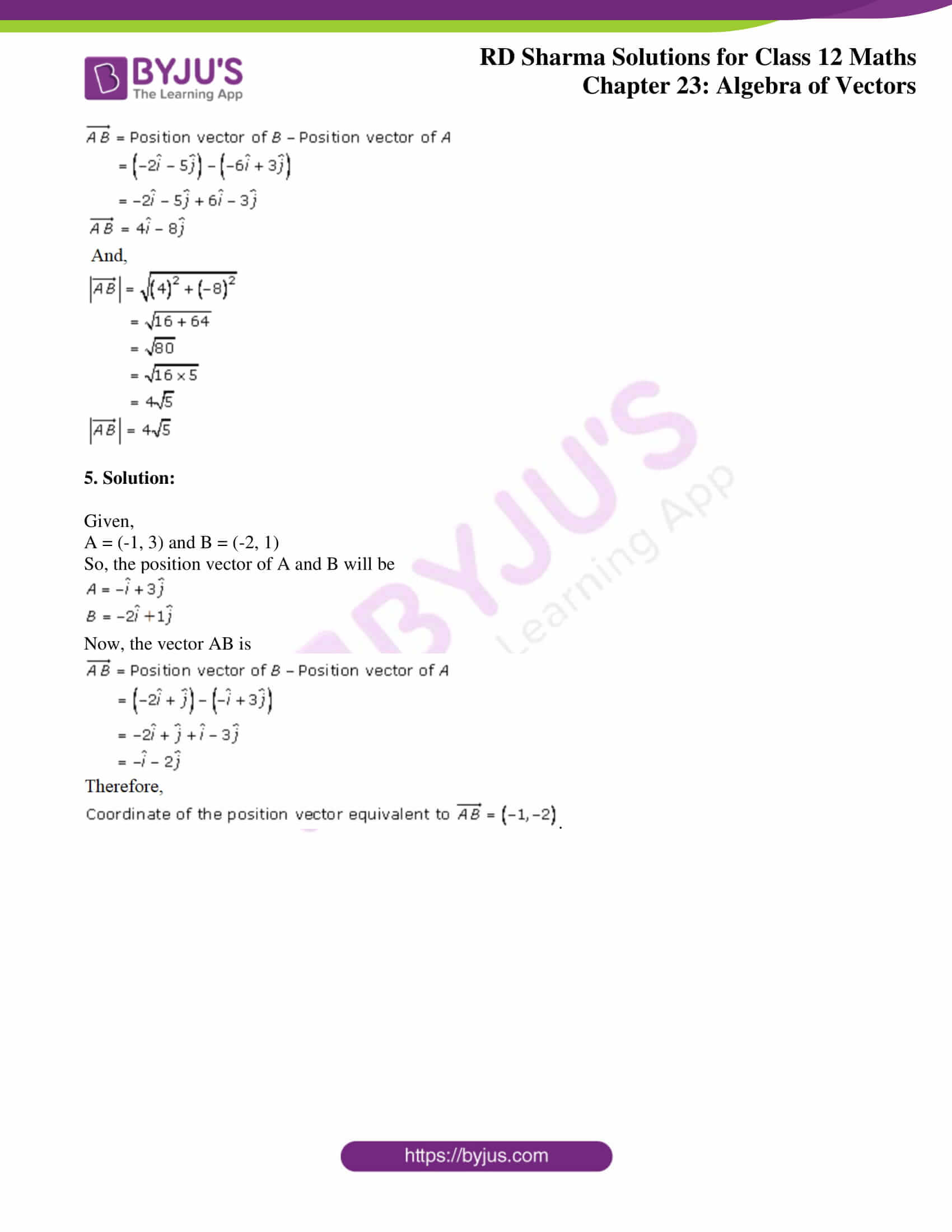### Access Answers for Rd Sharma Solution Class 12 Maths Chapter 23 Exercise 5

Exercise 23.5

1. Solution:

Given,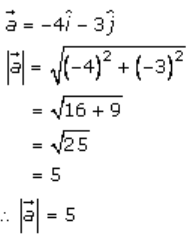2. Solution:

Given,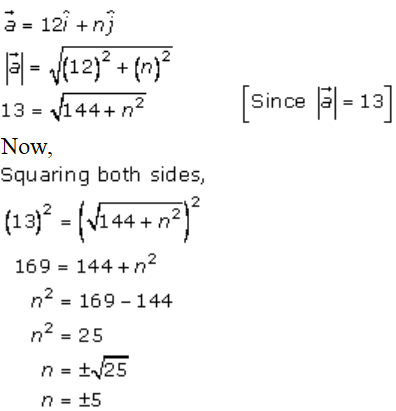3. Solution:

Given,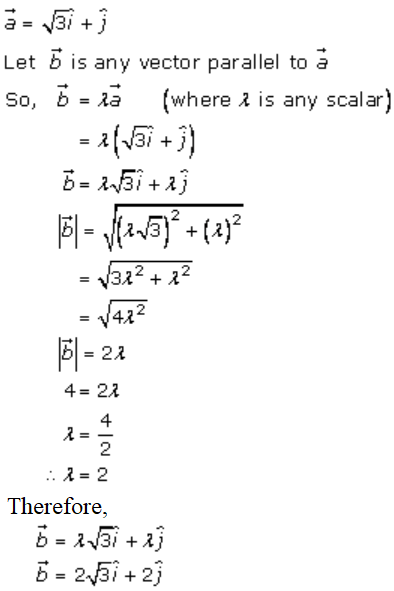4. (i)

Solution:

Given,

A = (4, -1) and B = (1, 3)

So, the position vector of A and B will be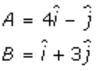Now, the vector AB is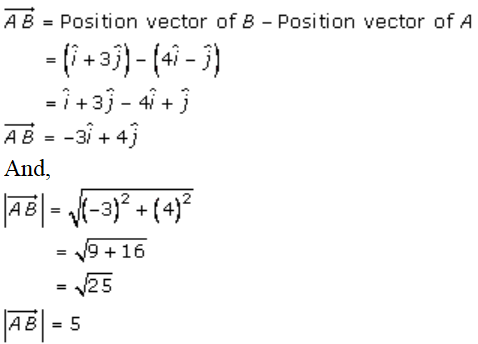4. (ii)

Solution:

Given,

A = (-6, 3) and B = (-2, -5)

So, the position vector of A and B will be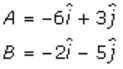Now, the vector AB is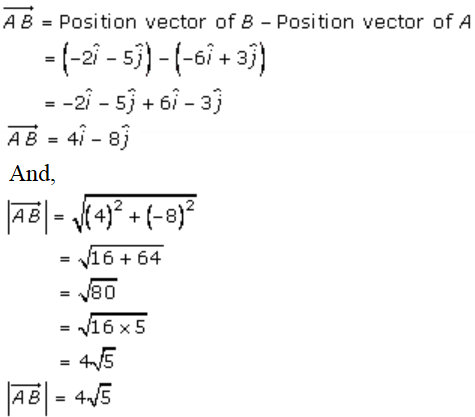5. Solution:

Given,

A = (-1, 3) and B = (-2, 1)

So, the position vector of A and B will be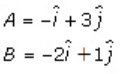Now, the vector AB is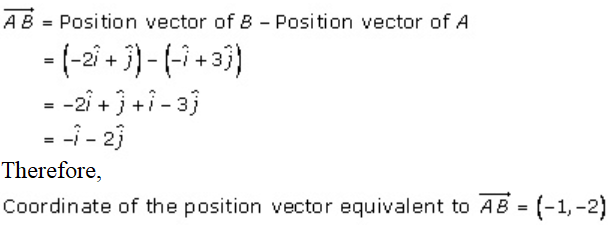.